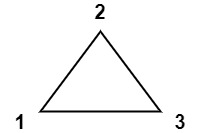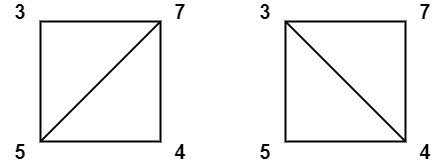Version: v1.6.5 Latest LatestGo to latest
Published: Mar 23, 2021 License: MIT

##README ¶

### 1039. Minimum Score Triangulation of Polygon (Medium)

You have a convex `n`-sided polygon where each vertex has an integer value. You are given an integer array `values` where `values[i]` is the value of the `ith` vertex (i.e., clockwise order).

You will triangulate the polygon into `n - 2` triangles. For each triangle, the value of that triangle is the product of the values of its vertices, and the total score of the triangulation is the sum of these values over all `n - 2` triangles in the triangulation.

Return the smallest possible total score that you can achieve with some triangulation of the polygon.

Example 1:```Input: values = [1,2,3]
Output: 6
Explanation: The polygon is already triangulated, and the score of the only triangle is 6.
```

Example 2:```Input: values = [3,7,4,5]
Output: 144
Explanation: There are two triangulations, with possible scores: 3*7*5 + 4*5*7 = 245, or 3*4*5 + 3*4*7 = 144.
The minimum score is 144.
```

Example 3:```Input: values = [1,3,1,4,1,5]
Output: 13
Explanation: The minimum score triangulation has score 1*1*3 + 1*1*4 + 1*1*5 + 1*1*1 = 13.
```

Constraints:

• `n == values.length`
• `3 <= n <= 50`
• `1 <= values[i] <= 100`

#### Hints

Hint 1 Without loss of generality, there is a triangle that uses adjacent vertices A and A[N-1] (where N = A.length). Depending on your choice K of it, this breaks down the triangulation into two subproblems A[1:K] and A[K+1:N-1].

##Documentation ¶There is no documentation for this package.